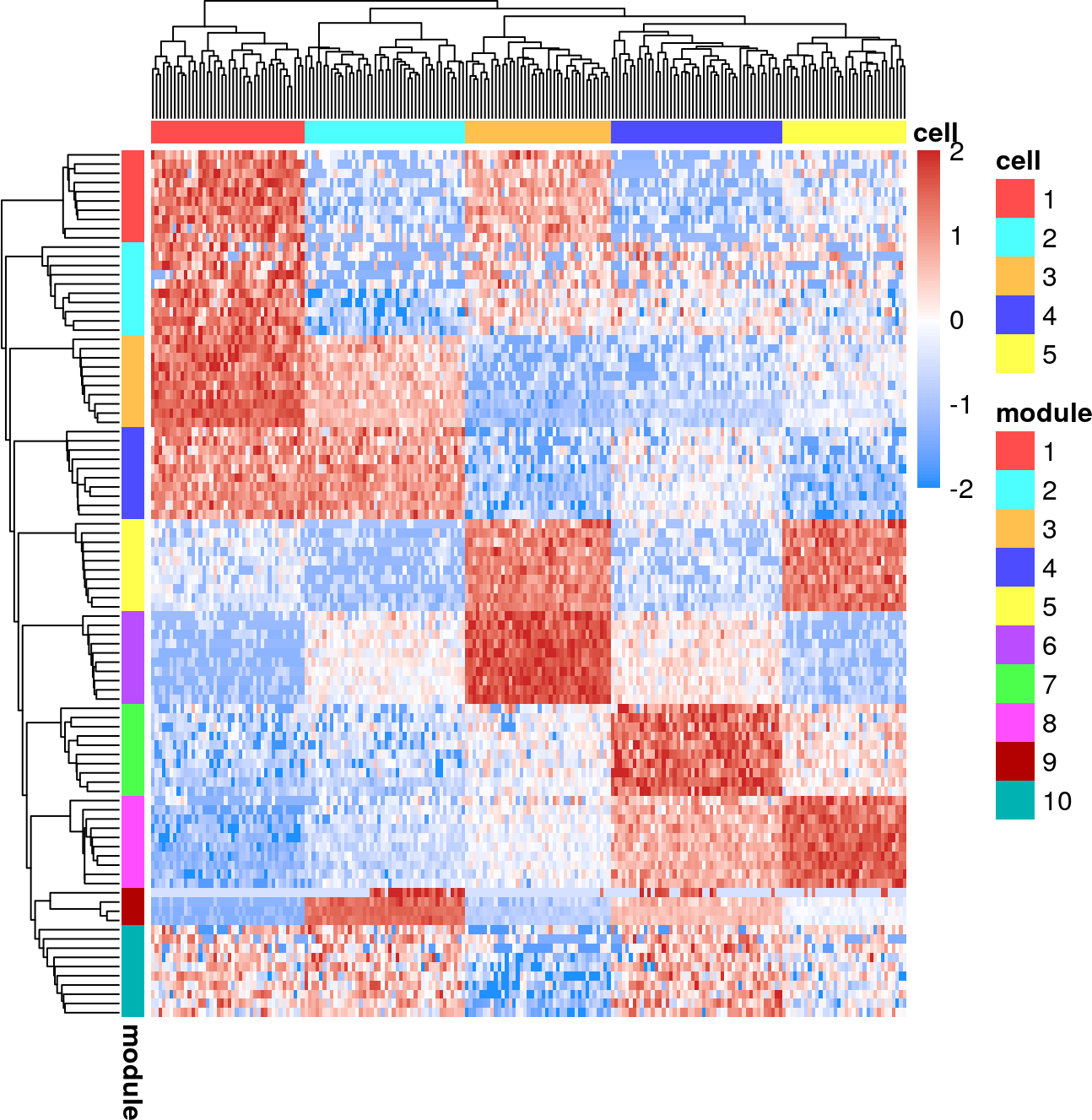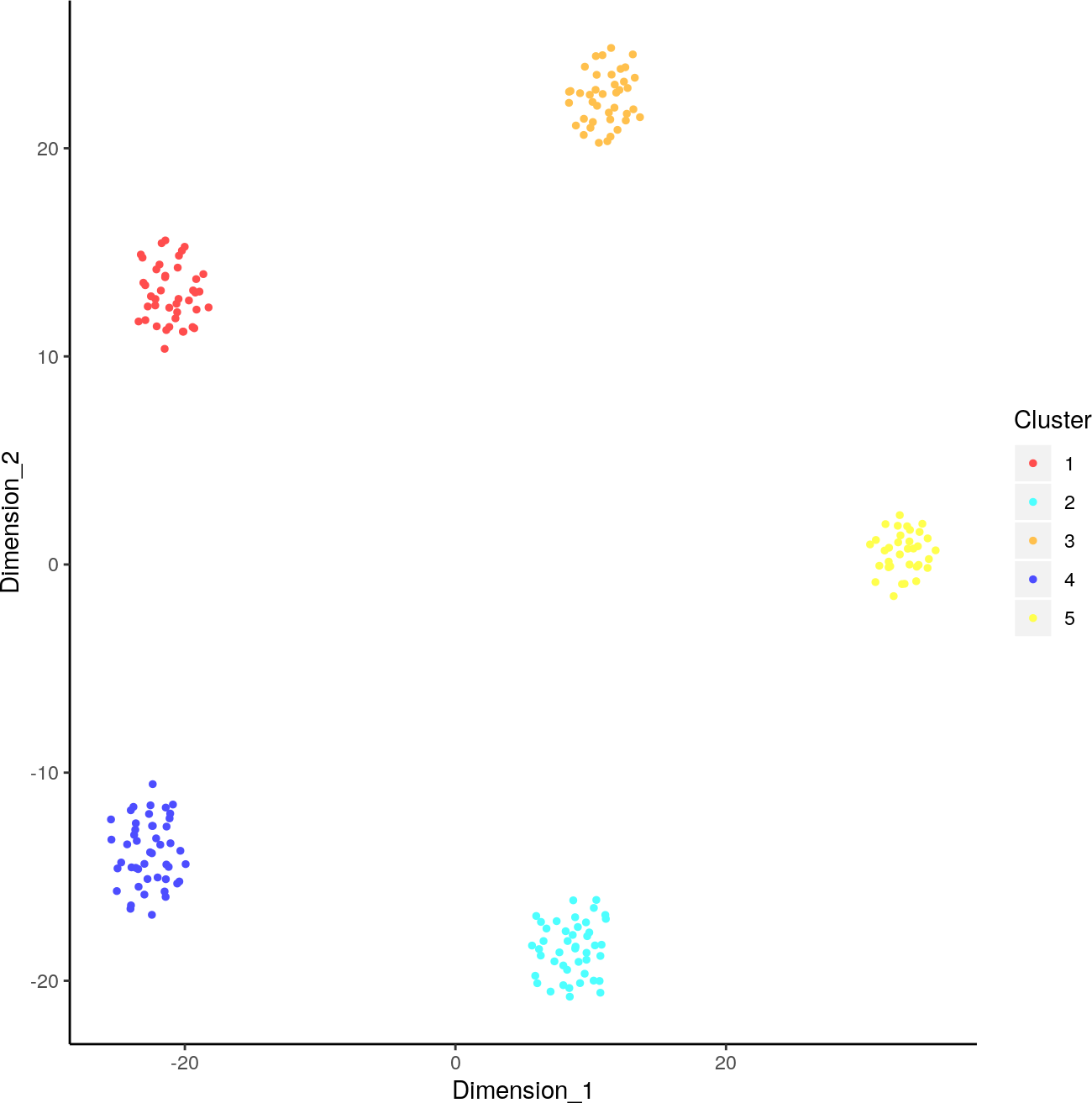# 1 Introduction

CEllular Latent Dirichlet Allocation (celda) is a collection of Bayesian hierarchical models to perform feature and cell bi-clustering for count data generated by single-cell platforms. This algorithm is an extension of the Latent Dirichlet Allocation (LDA) topic modeling framework that has been popular in text mining applications and has shown good performance with sparse data. celda simultaneously clusters features (i.e. gene expression) into modules based on co-expression patterns across cells and cells into subpopulations based on the probabilities of the feature modules within each cell. celda uses Dirichlet-multinomial distributions to model cells and genes so no additional normalization is required for single-cell counts.

In this vignette we will demonstrate how to use celda to perform cell and feature clustering with simulated data.

# 2 Installation

celda can be installed from Bioconductor:

``````if (!requireNamespace("BiocManager", quietly=TRUE)){
install.packages("BiocManager")}
BiocManager::install("celda")``````

The package can be loaded using the `library` command.

``library(celda)``

Complete list of help files are accessible using the help command with a `package` option.

``help(package = celda)``

To see the latest updates and releases or to post a bug, see our GitHub page at https://github.com/campbio/celda. To ask questions about running celda, post a thread on Bioconductor support site at https://support.bioconductor.org/.

# 3 Reproducibility note

Many functions in celda make use of stochastic algorithms or procedures which require the use of random number generator (RNG) for simulation or sampling. To maintain reproducibility, all these functions use a default seed of 12345 to make sure same results are generated each time one of these functions is called. Explicitly setting the `seed` arguments is needed for greater control and randomness.

# 4 Generation of a simulated single cell dataset

celda will take a matrix of counts where each row is a feature, each column is a cell, and each entry in the matrix is the number of counts of each feature in each cell. To illustrate the utility of celda, we will apply it to a simulated dataset.

In the function `simulateCells`, the K parameter designates the number of cell clusters, the L parameter determines the number of feature modules, the S parameter determines the number of samples in the simulated dataset, the G parameter determines the number of features to be simulated, and CRange specifies the lower and upper bounds of the number of cells to be generated in each sample.

``````simCounts <- simulateCells("celda_CG",
K = 5, L = 10, S = 5, G = 200, CRange = c(30, 50))``````

The `counts` variable contains the counts matrix. The dimensions of counts matrix:

``dim(simCounts\$counts)``
``##  200 207``

The `z` variable contains the cluster labels for each cell. Here is the number of cells in each subpopulation:

``table(simCounts\$z)``
``````##
##  1  2  3  4  5
## 42 44 40 47 34``````

The `y` variable contains the feature module assignment for each feature. Here is the number of features in each feature module:

``table(simCounts\$y)``
``````##
##  1  2  3  4  5  6  7  8  9 10
## 23 39 17 15 21 22 19 12  4 28``````

The `sampleLabel` is used to denote the sample from which each cell was derived. In this simulated dataset, we have 5 samples:

``table(simCounts\$sampleLabel)``
``````##
## Sample_1 Sample_2 Sample_3 Sample_4 Sample_5
##       43       48       45       40       31``````

# 5 Performing bi-clustering with celda

There are currently three models within this package: `celda_C` will cluster cells, `celda_G` will cluster features, and `celda_CG` will simultaneously cluster cells and features. Within the function the K parameter will be the number of cell populations to be estimated, while the L parameter will be the number of feature modules to be estimated in the output model.

``````celdaModel <- celda_CG(counts = simCounts\$counts,
K = 5, L = 10, verbose = FALSE)``````

Here is a comparison between the true cluster labels and the estimated cluster labels, which can be found within the `z` and `y` objects.

``table(clusters(celdaModel)\$z, simCounts\$z)``
``````##
##      1  2  3  4  5
##   1 42  0  0  0  0
##   2  0 44  0  0  0
##   3  0  0 40  0  0
##   4  0  0  0 47  0
##   5  0  0  0  0 34``````
``table(clusters(celdaModel)\$y, simCounts\$y)``
``````##
##       1  2  3  4  5  6  7  8  9 10
##   1   1 39  0  0  0  0  0  0  0  0
##   2  22  0  0  0  0  0  0  0  0  0
##   3   0  0 17  0  0  0  0  0  0  0
##   4   0  0  0 15  0  0  0  0  0  0
##   5   0  0  0  0 21  0  0  0  0  0
##   6   0  0  0  0  0 22  0  0  0  0
##   7   0  0  0  0  0  0 18  0  0  2
##   8   0  0  0  0  0  0  0 12  0  0
##   9   0  0  0  0  0  0  0  0  4  0
##   10  0  0  0  0  0  0  1  0  0 26``````

# 6 Matrix factorization

celda can also be thought of as performing matrix factorization of the original counts matrix into smaller matrices that describe the contribution of each feature in each module, each module in each cell population, or each cell population in each sample. Each of these following matrices can be viewed as raw counts, proportions, or posterior probabilities.

``````factorized <- factorizeMatrix(counts = simCounts\$counts, celdaMod = celdaModel)
names(factorized)``````
``##  "counts"      "proportions" "posterior"``

The `cell` object contains each feature module’s contribution to each cell subpopulation. Here, there are 10 feature modules to 207 cells.

``dim(factorized\$proportions\$cell)``
``##   10 207``
``head(factorized\$proportions\$cell[, seq(3)], 5)``
``````##        Cell_1      Cell_2     Cell_3
## L1 0.01709402 0.010452962 0.01117318
## L2 0.04957265 0.019163763 0.06331471
## L3 0.03076923 0.193379791 0.04841713
## L4 0.04957265 0.182926829 0.08193669
## L5 0.01367521 0.003484321 0.01675978``````

The `cellPopulation` contains each feature module’s contribution to each of the cell states. Since K and L were set to be 5 and 10, there are 5 cell subpopulations to 10 feature modules.

``````cellPop <- factorized\$proportions\$cellPopulation
dim(cellPop)``````
``##  10  5``
``head(cellPop, 5)``
``````##            K1          K2         K3         K4         K5
## L1 0.16530605 0.020828416 0.10820256 0.01485501 0.03701279
## L2 0.11236265 0.018261742 0.05533085 0.04767835 0.03708982
## L3 0.37986979 0.200820156 0.01931726 0.03993951 0.07837775
## L4 0.17846440 0.171554166 0.02556221 0.06609144 0.02391773
## L5 0.03107024 0.008703092 0.20944831 0.02232699 0.22758435``````

The `module` object contains each feature’s contribution to the module it belongs.

``dim(factorized\$proportions\$module)``
``##  200  10``
``head(factorized\$proportions\$module, 5)``
``````##        L1 L2 L3         L4 L5          L6 L7          L8 L9          L10
## Gene_1  0  0  0 0.01458423  0 0.000000000  0 0.000000000  0 0.0000000000
## Gene_2  0  0  0 0.00000000  0 0.000000000  0 0.000000000  0 0.0020778781
## Gene_3  0  0  0 0.00000000  0 0.005295658  0 0.000000000  0 0.0000000000
## Gene_4  0  0  0 0.00000000  0 0.000000000  0 0.000000000  0 0.0005862352
## Gene_5  0  0  0 0.00000000  0 0.000000000  0 0.008591603  0 0.0000000000``````

The top features in a feature module can be selected using the `topRank` function on the `module` matrix:

``````topGenes <- topRank(matrix = factorized\$proportions\$module,
n = 10, threshold = NULL)``````
``topGenes\$names\$L1``
``````##   "Gene_175" "Gene_20"  "Gene_180" "Gene_132" "Gene_112" "Gene_134"
##   "Gene_10"  "Gene_191" "Gene_32"  "Gene_90"``````

# 7 Visualization

## 7.1 Creating an expression heatmap using the celda model

The clustering results can be viewed with a heatmap of the normalized counts using the function `celdaHeatmap`. The top `nfeatures` in each module will be selected according to the factorized module probability matrix.

``celdaHeatmap(counts = simCounts\$counts, celdaMod = celdaModel, nfeatures = 10)``## 7.2 Plotting cell populations with tSNE

celda contains its own wrapper function for tSNE, `celdaTsne`, which can be used to embed cells into 2-dimensions. The output can be used in the downstream plotting functions `plotDimReduceCluster`, `plotDimReduceModule`, and `plotDimReduceFeature` to show cell population clusters, module probabilities, and expression of a individual features, respectively.

``tsne <- celdaTsne(counts = simCounts\$counts, celdaMod = celdaModel)``
``````plotDimReduceCluster(dim1 = tsne[, 1],
dim2 = tsne[, 2],
cluster = clusters(celdaModel)\$z)````````````plotDimReduceModule(dim1 = tsne[, 1],
dim2 = tsne[, 2],
celdaMod = celdaModel,
counts = simCounts\$counts,
rescale = TRUE)``````